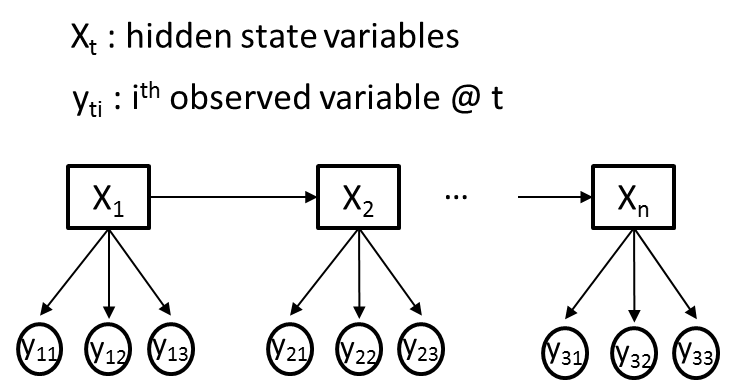2 views

I am trying to use a hidden Markov model (HMM) for a problem where I have M different observed variables (Yti) and a single hidden variable (Xt) at each time point, t. For clarity, let us assume all observed variables (Yti) are categorical, where each Yti conveys different information and as such may have different cardinalities. An illustrative example is given in the figure below, where M=3.My goal is to train the transition,emission and prior probabilities of an HMM, using the Baum-Welch algorithm, from my observed variable sequences (Yti). Let's say, Xt will initially have 2 hidden states.

I have read a few tutorials (including the famous Rabiner paper) and went through the codes of a few HMM software packages, namely 'HMM Toolbox in MatLab' and 'hmmpytk package in Python'. Overall, I did an extensive web search and all the resources -that I could find- only cover the case, where there is only a single observed variable (M=1) at each time point. This increasingly makes me think HMM's are not suitable for situations with multiple observed variables.

• Is it possible to model the problem depicted in the figure as an HMM?

• If it is, how can one modify the Baum-Welch algorithm to cater for training the HMM parameters based on the multi-variable observation (emission) probabilities?

• If not, do you know of a methodology that is more suitable for the situation depicted in the figure?

In this paper, the situation depicted in the figure is described as a Dynamic Naive Bayes, which -in terms of the training and estimation algorithms- requires a slight extension to Baum-Welch and Viterbi algorithms for a single-variable HMM.

by (33.1k points)

If you want to keep the model remain generative, then make the y_is conditionally independent to the given the x_is. This would lead to trivial estimators, but you should also take some restrictive assumptions in some cases.

For each timestep i, you have a multivariate observation y_i = {y_i1...y_in}. You can treat the y_ij as conditionally independent variable given x_i, so that:

p(y_i|x_i) = \prod_j p(y_ij | x_i)

A naive Bayes classifier for each possible value of the hidden variable x can be implemented. This can be learned with standard EM for an HMM.

For more details on Bayes' Theorem and Markov Model, study Machine Learning Algorithms and Machine Learning Tutorial.